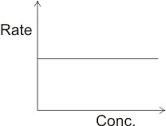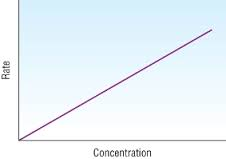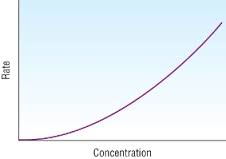# Kinetics- Rate of Reaction.

?
• Created by: Sophie
• Created on: 25-09-15 09:23

## Techniques to Measure Rates of Reactions.

Rate of reaction= change in concentration/change in time.

The rate of a reaction can be measured in many ways.

The volume of a gas produced can be measured, either over water (by counting the amount of bubbles produced, or more accurately the amount of water displaced) or using a gas syringe.

The change in mass of the reaction mixture. This is an easy technique as the only thing that is needed is sensitive scales.

Monitoring the colour change, using a colorimeter. The amount of time taken for the reaction to change colour is measured. Using a colorimeter is more accurate than using just the human eye.

Titrimetric analysis can be used by removing small portions of the reacting mixture and adding it to another reagent to quench the reation, the reaction is no longer going, acid has been neutralised but the concentrations have stayed the same. A titration is then carried out against the reactants.

Conductmetric analysis. The number and type of ions affect the conductivity of the solution and the change in conductivity of the solution is measured to measure the rate of the reaction.

1 of 10

## Factors Affecting the Rate of Reaction.

There are a range of different factors that affect the rate of reaction. These include:

• concentration- the more particles there are the more chance of a successful collisions.
• temperature- the higher the temperature is the more kinetic energy that can be transfarred to the particles so the more chance of successful collisions.
• pressure- (this is for gases only) the higher the pressure the closer the particles are together so the higher the chance of successful collisions.
• surface area- the larger the surface area the more chance that the particles have of hitting each other so the higher the chance of successful collisions.
2 of 10

## Rate Equation, Rate Constant and Order of Reaction

The rate equation

Rate=k[A]n[B]m

[A] and [B] refers to the concentration of the reactants, k is the rate constant and m and n are the order with respect to that reactant.

The rate equation can only be determined by experiments as it is the results of the experiment so the order of each reactant within the reaction.

rate= x2 order=first order

rate= x4 order=second order

rate= no change order= zero order

The indicies add up to give the overall order of that reaction. Any reactants that have a 0 order are left out of the rate equation.

The rate equation often shows the slowest step of the reaction. This step is called the rate determining step

3 of 10

## Calculating Rate From Experimental Data

From experimental data rate can be determined in different ways. Either by looking at a graph or the rate equation.

To find out the rate of a reaction from a graph then a gradient has to be calculated. A tangent is drawn from the origin and then the triangle that is created from the long straight line is the area that the gradient is calculated from as it is the area with the most accurate results.

Using the rate equation to find out the rate just means that the data that is known needs to be plugged into the equation. It is often k that needs to be worked out find using known data to find out the rate of another repeat within the experiment. This is just done be rearranging the rate equation.

Rate=k[A]n[B]m

The rate constant (k) is dependent on temperature. Therefore, the number calculated will only be the rate constant for the reaction carried out at that temperature.

4 of 10

## Zero Order Graphs

The typical shape of a zero order concentration against time graph.The typical shape of a zero order rate against concentration graph.5 of 10

## First Order Graphs

The typical shape of a first order concentration against time graph.The typical shape of a first order rate against concentration graph.6 of 10

## Second Order Graphs

The typical shape of a second order concentration against time graph.The typical shape of a second order rate against concentration graph.7 of 10

## Catalysts

Catalysts speed up a reaction by offering an alternative pathway with a lower activation energy.

Activation energy- the lowest amount of energy that a molecule has to have in order for the reaction to take place. The higher it is the slower or less likely the reaction.

There are two different types of catalyst. homogeneous catalysts and heterogeneous catalysts.

Homogenous catalysts are in the same phase as the reactants. Such as a liquid catalysts in with liquid reactants or a gas catalyst with gas reactants.

Heterogeneous catalysts are in different phase to the reactants. Such as a solid catalyst being used with gases. They are most frequently transittion metals and the reacting materials are usually absorbed onto the surface of the catalyst to give a large surface area and miximise the rate of reaction.

8 of 10

## Activation Energy

Activation energy- the lowest amount of energy that a molecule has to have in order for the reaction to take place. The higher it is the slower or less likely the reaction.

Using kinetic theory and the probability theory the amount of molecules with a greater amount of energy than the activation energy can be shown. It is given by eEa/RT, where R is the gas constant, T is the absolute temperature and e is the exponential constant.

Therefore, at a given temperature:

Rate of reaction is equal to eEa/RT or it can be written as k=AeEa/RTas k is the rate constant. The A is Arrhenius constant. This applies natural logarithms. In k= -(Ea/R) + a constant.

A straight line can be represented by y= mx + c. So plotting a graph of In k (y) against 1/T (x) gives a straight graph whose gradient (m) is -(Ea/R). From this value you can work out the Ea using the R value of 8.314KJ-1mol-1.

9 of 10

## Calculating Units

Using the rate equation the units of each component are put in and then cancled out in order to get the final units of the answer from the equation.

E.g.

k= rate/[A]2[B]

moldm-3S-1/(moldm-3)2 moldm-3

The moldm-3 on the top and the bottom cancel each other out so they are removed.

S-1/(moldm-3)2

The bottom is squared to get rid of the brackets

S-1/mol2dm-6

The bottom is then divided by itself to put it on top of the equation to give the final units.

S-1mol-2dm6

10 of 10